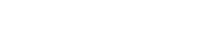# Certified Automation Professional (CAP) program

• CAP

## CAP question

In plant operations cost analysis, which of the following describes a “break-even” cost situation?

A. fixed costs = equipment costs + building cost + land cost
B. revenue = fixed costs + variable costs
C. total costs = fixed costs + variable costs
D. revenue > fixed costs – variable costs

The answer is B, “revenue = fixed costs + variable costs.” When the sum of all fixed costs and variable costs are added together, if the sum just equals the revenues (or expected revenues) received, then we say we are at a “break even” point.

If revenues are greater than the sum of the costs, then we are operating at a profit. If revenues are less than the sum of the costs, then we are operating at a loss.

Reference: Sands, Nicholas P. & Verhappen, Ian, A Guide to the Automation Body of Knowledge, Third Edition, ISA Press, 2019.